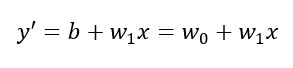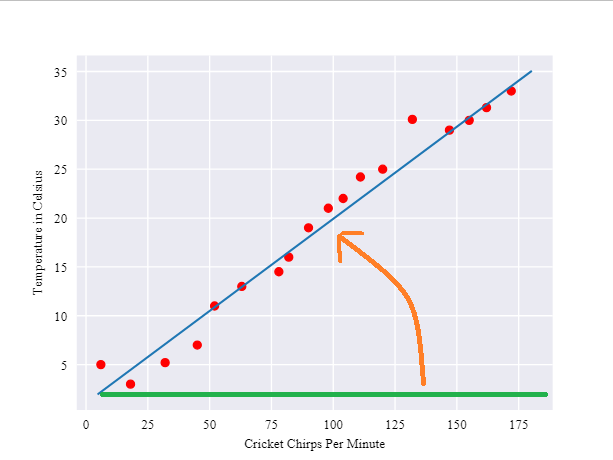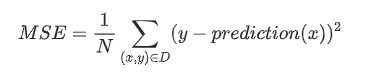2019 iT 邦幫忙鐵人賽DAY 5
0
AI & Data

[Day 5] 陷入Machine Learning之中

Reference: Descending into MLLearning的過程，大概長下面這樣，橘線是我們的初始值，會慢慢learning到藍色的線:y = mx + b

y: 溫度(要預測的項目)
m: 最後這條線的斜率
x: 蟋蟀叫聲次數
b: y截距(一點偏移量)

OK，這樣就把溫度、蟋蟀叫聲次數跟二元一次方程式湊起來了，剩下bm，再讓我們改寫一下:Squared loss
= Label 跟 prediction 相差的平方
= (observation - prediction(x))^2
= (y - y')^2

Mean square error (MSE) 則是把所有dataset裡的squared loss相加後平均(x, y): 代表每個example中的features x及其對應的label y
prediction(x): 代表權重w與偏移量b對每個x集合算出來的結果
D: 包含(一個、一部、或全部)labeled examples 的 dataset
N: dataset D的數量

Although MSE is commonly-used in machine learning, it is neither the only practical loss function nor the best loss function for all circumstances.